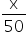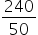Mathematics
Easy

Question

# There are 240 seniors in Kathryn’s school. Her class is planning a trip and is taking buses that hold a maximum of 50 passengers. Assume that the trip is optional. Write a step function f that maps the number of students x, to the number of buses needed, f(x).

## f(x) = 50 ceiling (x) , 0 < x ≤ 50 f(x) = ceiling () , 0 < x ≤ 50f(x) = floor () , 0 < x < 50f(x) = 50 floor () , 0 < x ≤ 50Hint:

## The correct answer is: f(x) = ceiling () , 0 < x ≤ 50

### The function is: f(x) = ceiling(), 0 < x ≤ 50 x = no of seniors, f(x) = No of buses needed.If x = 240, f(x) = ceiling() = ceiling(4.8) = 5In case of 240 passengers, 5 buses are needed.Hence, the correct option is A.

The ceiling function is also known as the smallest integer function. The floor function is also known as the greatest integer function.

### Related Questions to study#### With Turito Foundation.#### Get an Expert Advice From Turito.